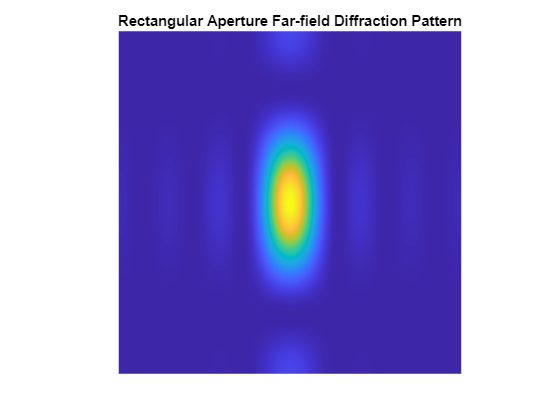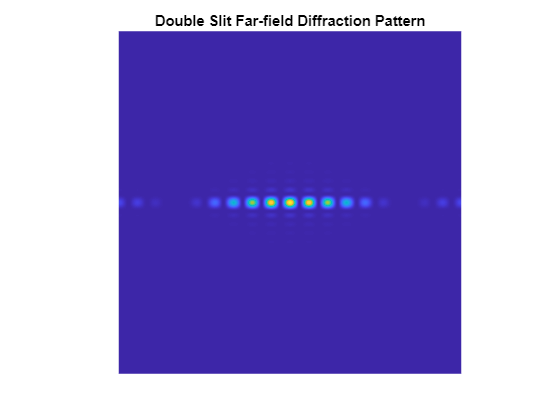# Using FFT2 on the GPU to Simulate Diffraction Patterns

This example uses Parallel Computing Toolbox™ to perform a two-dimensional Fast Fourier Transform (FFT) on a GPU. The two-dimensional Fourier transform is used in optics to calculate far-field diffraction patterns. These diffraction patterns are observed when a monochromatic light source passes through a small aperture, such as in Young's double-slit experiment.

### Defining the Coordinate System

Before we simulate the light that has passed through an aperture, we must define our coordinate system. To get the correct numerical behavior when we call `fft2`, we must carefully arrange `x` and `y` so that the zero value is in the correct place.

`N2` is half the size in each dimension.

```N2 = 1024; [gx, gy] = meshgrid( gpuArray.colon( -1, 1/N2, (N2-1)/N2 ) ); ```

### Simulating the Diffraction Pattern for a Rectangular Aperture

We simulate the effect of passing a parallel beam of monochromatic light through a small rectangular aperture. The two-dimensional Fourier transform describes the light field at a large distance from the aperture. We start by forming `aperture` as a logical mask based on the coordinate system, then the light source is simply a double-precision version of the aperture. The far-field light signal is found using `fft2`.

```aperture = ( abs(gx) < 4/N2 ) .* ( abs(gy) < 2/N2 ); lightsource = double( aperture ); farfieldsignal = fft2( lightsource ); ```

### Displaying the Light Intensity for a Rectangular Aperture

We calculate the far-field light intensity from the magnitude squared of the light field. Finally, we use `fftshift` to aid visualization.

```farfieldintensity = real( farfieldsignal .* conj( farfieldsignal ) ); imagesc( fftshift( farfieldintensity ) ); axis( 'equal' ); axis( 'off' ); title( 'Rectangular aperture far-field diffraction pattern' ); ```### Simulating Young's Double-Slit Experiment

One of the most famous experiments in optics is Young's double-slit experiment which shows light interference when an aperture comprises two parallel slits. A series of bright points is visible where constructive interference takes place. In this case, we form the aperture representing two slits. We restrict the aperture in the `y` direction to ensure that the resulting pattern is not entirely concentrated along the horizontal axis.

```slits = (abs( gx ) <= 10/N2) .* (abs( gx ) >= 8/N2); aperture = slits .* (abs(gy) < 20/N2); lightsource = double( aperture ); farfieldsignal = fft2( lightsource ); ```

### Displaying the Light Intensity for Young's Double-Slit

We calculate and display the intensity as before.

```farfieldintensity = real( farfieldsignal .* conj( farfieldsignal ) ); imagesc( fftshift( farfieldintensity ) ); axis( 'equal' ); axis( 'off' ); title( 'Double slit far-field diffraction pattern' ); ```Watch now